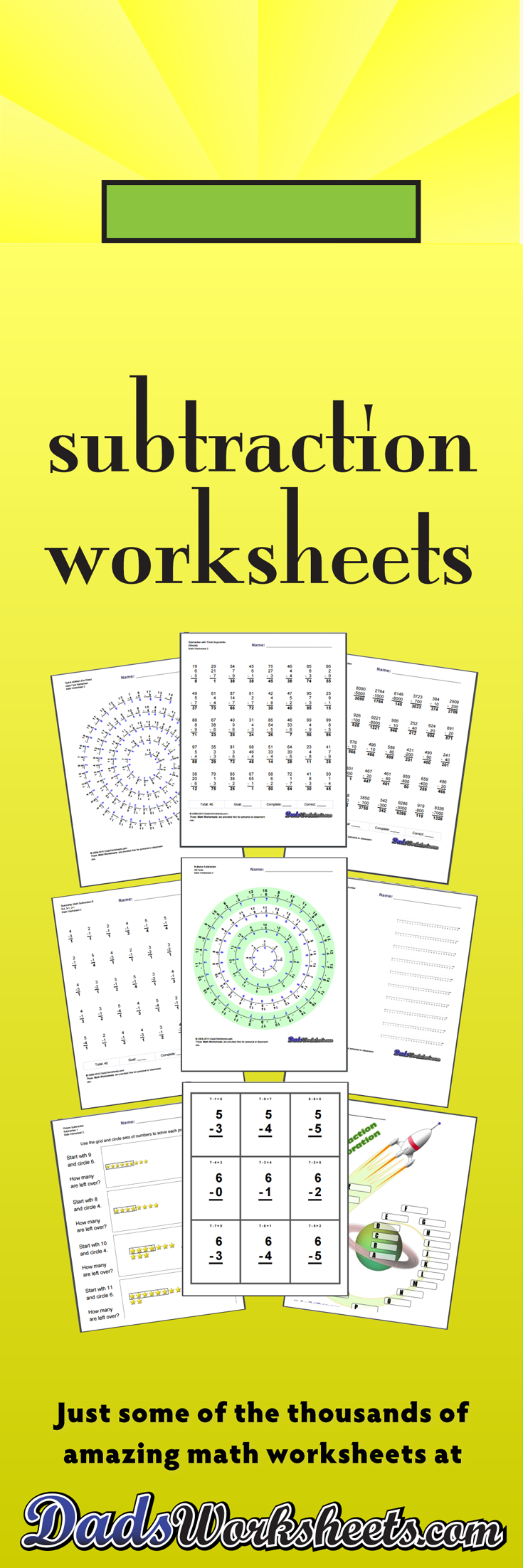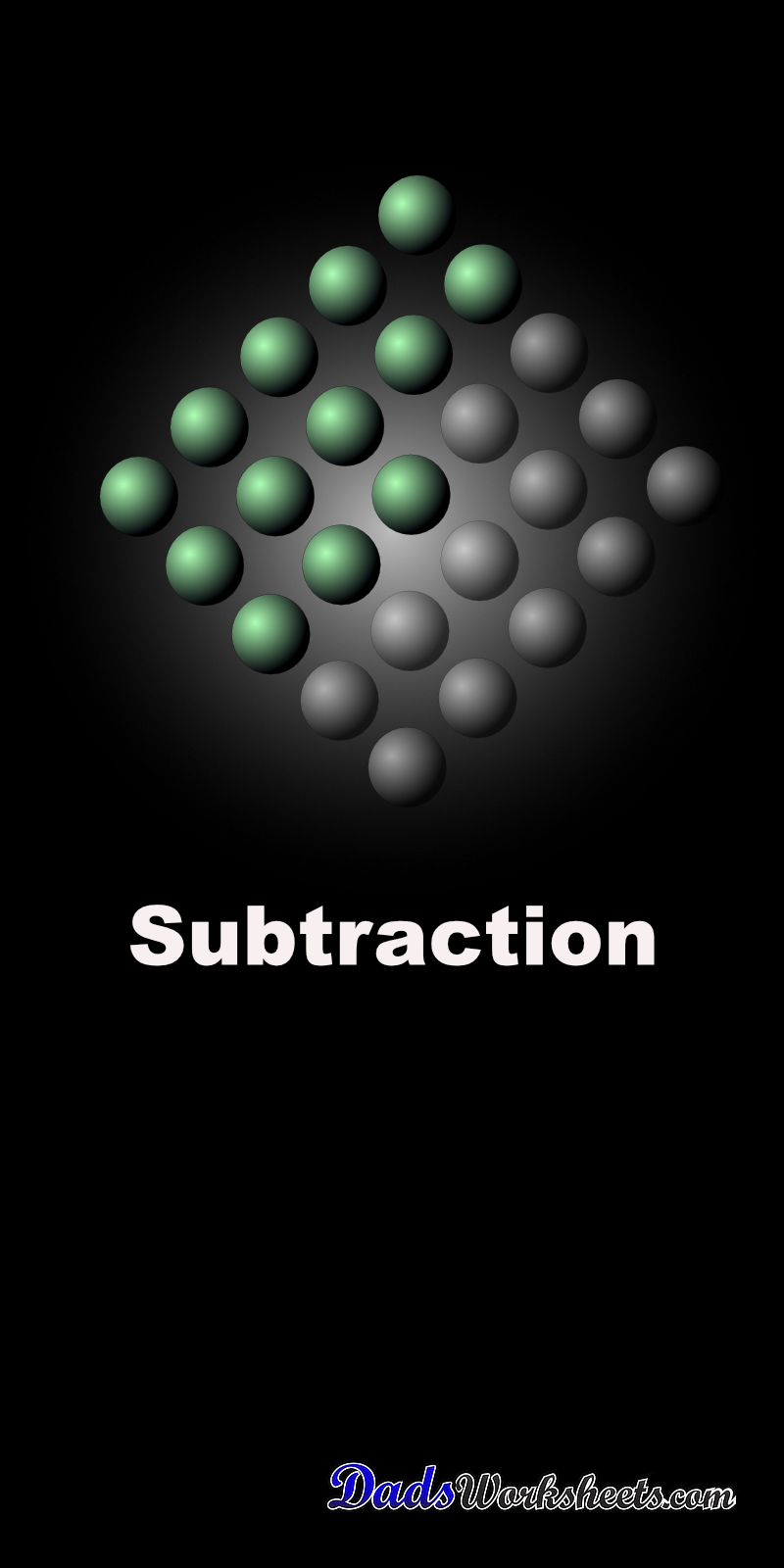# Subtraction Worksheets

These subtraction worksheets include timed math fact tests, multiple digit problems, subtraction with and without regrouping and much more. If you're looking for an incremental path from subtraction facts through basic multiple digit subtraction problems all the way to problems that are super-focused on subtraction regrouping, you've found everything you need! Try out the unique spiral and bullseye subtraction fact worksheets to get a completely different spin on your math fact practice. If I missed anything subtraction-related, please let me know!

## Subtraction with the Number Line

### 8 Subtraction Worksheets 515

These simple subtraction worksheets introduce subtraction concepts using the number Line.

Subtraction with the Number Line

## One Minute Timed Subtraction Worksheets

### 104 Subtraction Worksheets 516

The subtraction worksheets in this section are similar to the Mad Math Minutes or Mastering Math Facts subtraction worksheets used at many schools. These are typically one minute, timed subtraction tests. Try completing them with my super entertaining online timer if you or your kids are into silly sounds!

Spaceship Math

## Two Minute Tests

### 64 Subtraction Worksheets 517

These are two minute versions of the Spaceship Math subtraction worksheets with 80 and 100 problems per page.

Two Minute Tests

## Circle Subtraction Facts Worksheets

### 24 Subtraction Worksheets 518

These Subtraction worksheets emphasize groups of related facts and there are variations with the facts in order to facilitate skip counting, or with random products that help facilitate fact memorization. Try the variations with all facts, or print the worksheets that focus only on specific families of multiplication facts that need more practice!

Subtraction Fact Circles

## Spiral Subtraction Facts

### 56 Subtraction Worksheets 519

Tired of the same old math fact worksheets with rows and rows of problems? Time to try something unique. These worksheets present the subtraction facts in a spiral layout that provides fun a twist on memorizing the basic math facts. They use the same fact layouts as the spaceship math sheets above, so try the first two sets worksheets if you are looking for the full set of subtraction facts or practice without the easier problems, or look at the others for an incremental approach to learning everything related to the trusty minus sign!

Spiral Subtraction Facts

## Subtraction Sequence Worksheets

### 8 Subtraction Worksheets 520

These subtraction worksheets allow students to practice simple sequences of subtraction to arrive at a final answer to a problem. The worksheets start with very small differences and progress through multi-digit subtraction. There are also worksheets that mix addition and subtraction steps in the sequences for practicing both of these arithmetic skills together. They are a fun alternative to simple subtraction problems that keep the subtraction skills moving along!

Sequence Subtraction Worksheets

## Bullseye Subtraction

### 8 Subtraction Worksheets 521

Get on target with those subtraction math facts! These fun worksheets provide some additional kinesthetics over and above your usual math fact practice. These are great a great change to use as timed tests. Hopefully you will find these subtraction worksheets are spot-on when you need a change of pace!

Bullseye Subtraction

## Place Value

### 8 Subtraction Worksheets 522

Place value subtraction worksheets. These primary worksheets are a good lead into the multiple digit subtraction worksheets.

Place Value

## No Borrowing

### 24 Subtraction Worksheets 523

These multi-digit subtraction worksheets introduce problems without borrowing or regrouping.

No Borrowing

## Borrowing Without Borrowing Across a Zero

### 24 Subtraction Worksheets 524

These worksheets are the next step in learning multiple digit subtraction. These multiple digit subtraction worksheets introduce borrowing (regrouping), but not borrowing across a zero.

Borrowing Without Borrowing Across a Zero

## Borrowing Across Zero

### 24 Subtraction Worksheets 525

These subtraction worksheets focus on subtraction incorporating borrowing across zeroes. These are great subtraction worksheets to use to really practice borrowing if your student is struggling with regrouping concepts.

Borrowing Across Zero

## Full Borrowing

### 44 Subtraction Worksheets 526

Multiple Digit Subtraction worksheets with a more conventional mix of problems (includes borrowing/regrouping.) See the worksheets in prior sections for more focused subtraction topics introducing multiple digits.

Full Borrowing

## Subtraction with Decimals

### 24 Subtraction Worksheets 527

Multi-digit subtraction worksheets that introduce decimals. This section includes sets of worksheets including subtraction with tenths, subtraction with hundredths and subtraction with thousandths.

Subtraction with Decimals

## Three Argument Subtraction

### 16 Subtraction Worksheets 528

Subtraction worksheets with three arguments.

Three Argument Subtraction

### 16 Subtraction Worksheets 529

Math worksheets with mixed addition and subtraction problems. These problems help a lot to get students to pay attention to operations.

## Introduction to Negative Numbers

### 16 Subtraction Worksheets 530

Simple subtraction worksheets showing negative answers introduced with the number line. This is your starting point for negative numbers.

Introduction to Negative Numbers

## Subtraction with Negative Results

### 28 Subtraction Worksheets 531

Practice worksheets with subtraction problems that may yield negative answers.

Subtraction with Negative Results

## Subtraction Worksheets for Math Practice!

Have you mastered the addition facts? Time to move on to subtraction! These subtraction fact timed tests and multiple digit problems introduce increasingly complex topics such as regrouping (borrowing/carrying) and you'll find plenty of multiple digit subtraction practice worksheets here to keep young minds engaged, but when you're ready for even more, be sure to check out these other math worksheets: